• Call Now

1800-102-2727•

# Power factor, Apparent power, True power, reactive power, practice problems, FAQs

Let me tell you one interesting fact. Why do you think there are different energy metres for domestic and industrial use? For domestic use we only pay for the amount of power we have used. But in the case of industrial purposes there are extra charges if the power factor is not maintained above a certain level. We will discuss the term the power factor in detail. If the power factor is low then a huge amount of power gets wasted. In this article we will be studying how power is calculated in AC circuits and its different types.

• Instantaneous power in AC circuit
• Average power in AC circuit
• Real power
• Apparent power
• Power factor
• Practice problems
• FAQs

## Instantaneous power in AC circuit

The main benefit of alternating current is that it is simple to convert AC voltages from higher to lower voltage levels and vice versa. High-voltage power from power plants can be reduced to a safer voltage for household usage because of this property. Capacitors and inductors only work with alternating current. By using them within the Alternating current circuits, the flow of electricity can be turned. This property helps tune the radio stations. AC power is favoured for residential appliances because of these factors.

Let voltage V is applied to the AC circuit and it flows a current I through it.

V=Vmsin(t)

I=Imsin(t+)

Here, Vm and Im are peak values of voltage and current respectively.

is the angular frequency and is phase constant.

Instantaneous power, Pinst=VI

Pinst=Vmsin(t)Imsin(t-)

By using, 2 sinA sinB = cos(A − B) − cos(A + B)

Pinst=VmIm2[cos()-cos(2t-)]

If we plot this we get following graph,From the graph we can observe that power is varying sinusoidally. So both current and voltage are positive or negative then the instantaneous power is positive. The positive instantaneous power means that power is being supplied by the source to the circuit. On the other hand, if any one from current or voltage is negative then instantaneous power is negative. Negative instantaneous power means that power is being absorbed by the source from the circuit.

This equation demonstrates that instantaneous AC power is divided into two parts and is hence the sum of the two terms. Due to the 2 components of the term, the second term is a time variable sinusoid with a frequency equal to twice the angular frequency of the supply. However, the first term is a constant whose value is solely determined by the phase difference between the voltage (V) and the current (I). Let’s see two cases when current and voltage are in phase and out of phase by 900.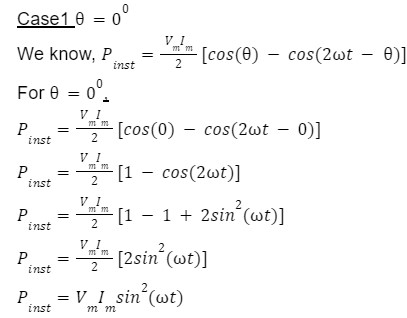From the above equation we can see that instantaneous power will never be negative in this case. That means the source will keep supplying power to the circuit. This is the special case when the circuit is purely resistive.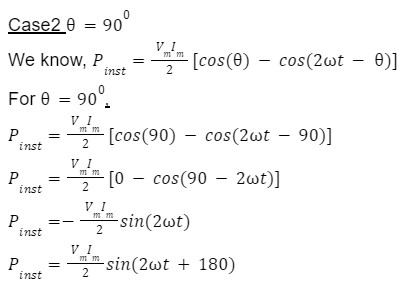In this case instantaneous power is varying sinusoidally with frequency twice of source frequency and 1800 out of phase.

## Average power in AC circuit

It's tough to estimate instantaneous power because it's continually changing with the sinusoid's profile throughout time. Using the average or mean value of the power is consequently more convenient and easier on the maths.

We know, instantaneous power from above equation,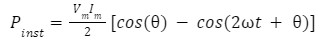Now to calculate the average power we have to calculate the average value of instantaneous power,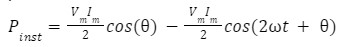So the first term in the above equation is constant so its average value will be the same as itself. But the second term is varying sinusoidally so its average value will be zero. So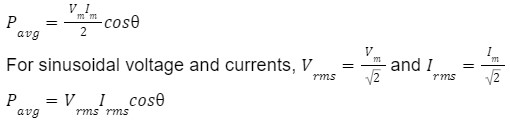## Apparent Power

It is the total power i.e. combination of real power and reactive power in an AC circuit. It is measured in VA (Volt Ampere)Let voltage V is applied to the AC circuit and it flows a current I through it.

V=Vmsin(t+V)

I=Imsin(t+I)

Here, Vm and Im are peak values of voltage and current respectively.

Complex power is defined as,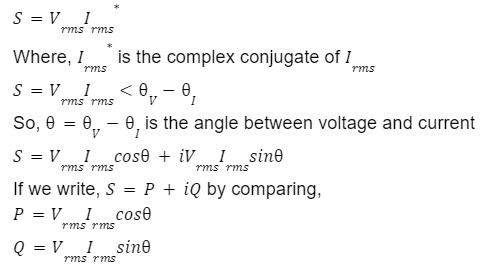Active Power: The power which is actually consumed or utilised in an AC Circuit is called True power or Active Power or real power. P is called active power in the above equation. Active power is the real component of complex power. It is measured in watts.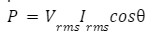Reactive Power: A Power which continuously bounces back and forth between source and load is known as Reactive Power. (Also known as watt-less power or useless power.). Q is called reactive power in the above equation. It is measured in VAR (Volt Ampere Reactive). It is the imaginary component of complex power.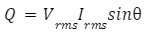## Power factor

Power Factor (PF) is an indicator of efficient utilisation of power. In an AC circuit, PF is defined as the ratio of real power flowing to the load, to the apparent power in the circuit and is a dimensionless number.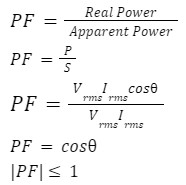So the power factor is the cosine of angle between voltage and current flowing through the AC circuit.

Unity Power Factor

If the power factor is unity that means cos=1, that implies =00, is the phase difference between voltage and current in an AC circuit. So at unity power factor current and voltage are in phase. This is the special case when the circuit is purely a resistive or reactive circuit under resonance. At unity power factor there is no reactive power in the circuit so whole power is in the form of real power.

## Practice problems

Q 1. Two loads of 10 KW each, are operating at a power factor 0.8 lagging (each).What is their combined power factor?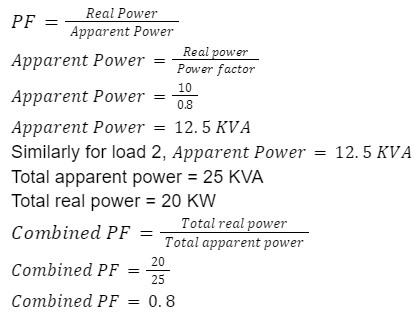Q 2. v(t)=240 sin(t+60) Volt and i(t)=5sin(t-10) A are the voltage and current values of a 50 Hz sinusoidal source, respectively. Find the value of instantaneous power ?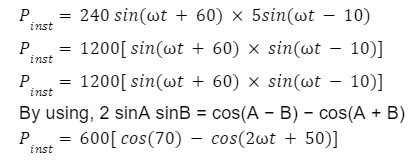Q 3. Find the average power if voltage and current is the same as the previous question?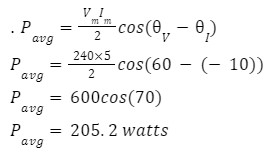Q 4. Find apparent power in the AC circuit if real power is 30 Kw and reactive power is 40 KVAR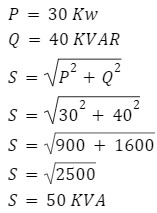## FAQs

Q 1. What causes the reactive power?
Reactive power is either generated or absorbed by electric generators (in some cases capacitors) to maintain a constant voltage level.

Q 2. Where reactive power is required?
Reactive power is used by most types of electrical equipment that uses a magnetic field, such as motors, generators and transformers.

Q 3. What is the distinction between reactive and active power?
Active power is the power that continuously flows from source to load in an electric circuit. Reactive power is the power that continuously flows from source to load and returns back to source in an electric circuit.

Q 4. What is the significance of the power factor?
The significance of the power factor lies in the fact that utility companies supply customers with Apparent power (VA), but bill them for real power (watts). Power factors below 1.0 require a utility to generate more than the minimum VA necessary to supply the real power (watts). This increases generation and transmission costs.Talk to our expert
Resend OTP Timer =
By submitting up, I agree to receive all the Whatsapp communication on my registered number and Aakash terms and conditions and privacy policy